# Math Resources: Middle, Secondary, Post-Secondary Subject Resources And Apps for Mobile DevicesThis collection of Math Resources has multiple pages designed for teaching within specific grade bands.  You'll also find valuable collections of support and enrichment resources that will benefit all learners.

Math Resources (Page 1): K-8 Basic Mathematics and Skills Development, plus apps for mobile devicesMath Resources (Page 2): Middle, Secondary, Post-Secondary Subject Resources: Subject Specific Resources also include apps for your mobile devices:

Math Resources (Page 3): K-12 Supplementary Collections: Miscellaneous Math Collections; Practical Applications--Math in Careers, Daily Life, and Across the Curriculum; and Problem Solving

Math Resources (Page 4): Enrichment and Extra Help: Math Contests, Competitions, Challenges, and Camps; Study Skills and Homework Help, plus tips for parent involvement; and Dictionaries, Glossaries, Reference Sheets, and Math Encyclopedias

Math Resources (Page 5): Lesson Plans and Worksheets, Design and Manage Your Own Lesson Plans, and Publishers' Textbook Support Sites

Math Resources (Page 6): Resources for Podcasts and Vodcasts, the Flipped Learning Model, and for Whiteboards, including tips on using whiteboards## Middle, Secondary, Post-Secondary Subject Resources

In addition to the subject resources presented in this section, see our Common Core section for specific strand resources for number and quantity, algebra, functions, geometry, and statistics and probability.  Each domain includes technology investigations, multiple choice and constructed response exercises, and performance tasks related to each Common Core high school math standard.  These will benefit all learners in any state.

### Handbook of Essential Mathematics

Teachers: You and your students might come to value the Handbook of Essential Mathematics from the Air Force Research Laboratory (2006) at Wright Patterson Air Force Base in Dayton, Ohio.  It's particularly relevant for subjects addressed on this page of resources.  This free online handbook is open source and has three sections "that will well serve both students and teachers alike from early grades through early college" (p. 3).  Those are described as follows:

"Section I, “Formulas”, contains most of the mathematical formulas that a person would expect to encounter through the second year of college regardless of major. In addition, there are formulas rarely seen in such compilations, included as a mathematical treat for the inquisitive. Section I also includes select mathematical processes, such as the process for solving a linear equation in one unknown, with a supporting examples. Section II, “Tables”, includes both ‘pure math’ tables and physical-science tables," including some "just to nurture curiosity in a spirit of fun." ... Section III, “Applications in Personal Finance”, applies the language of algebra to everyday finances.  (p. 4)

#### Need a quick review?

HOT!: eCalc Math Help Reference Sheets for algebra, geometry, trigonometry (definitions, laws, identities), and calculus (derivatives, limits, integrals) provide a quick review.  There are six reference sheets in all with key definitions, properties, operations, and formulas in each subject, all in one file.

## Algebra and Pre-Algebra

### What is Algebra?### Are you ready for algebra?Students: Find out by taking an Algebra Readiness Test.  This test at algebra-class.com includes answers, and content to review theory.

The following Dr. Math books (2003) from The Math Forum will help you, too.

Educators: In Foundations for Success, the National Mathematics Advisory Panel (2008) set an expectation for school algebra that "students to be able to proceed successfully at least through the content of Algebra II" (p. xvii).  Major topics recommended for school algebra include:

1. Symbols and Expressions: polynomial and rational expressions, arithmetic and finite geometric series.
2. Linear Equations, including linear inequalities
4. Functions: linear, quadratic, polynomial, simple non-linear, exponential, logarithmic, trigonometric, and fitting simple mathematical models to data
5. Algebra of Polynomials.  This study would also extend to include complex numbers and operations, and mathematical induction.
6. Combinations and permutations, as applications of the binomial theorem and Pascal’s Triangle, and Finite Probability. (p. 16).

Educators might be interested in the Ohio Algebra I Blueprint, which is helpful for planning curriculum and guiding instruction.  It includes the standards for number, quantities, equations and expressions, functions, and statistics typical for an algebra 1 course.  These standards are eligible to appear in the state year-end standardized test for algebra 1.HOT: AlgebraByExample "gives students practice solving problems and practice modeling, analyzing, critiquing, and articulating mathematical arguments."   There are 42 individual assignments within nine algebra 1 topics, including one for pre-algebra.  The assignments support the Common Core Practice Standards.  Problems are designed to address student misconceptions.  Each assignment contains a worked example accompanied by an analysis question.  Then learners solve a similar problem on their own.  The sets are free downloads from the SERP (Strategic Education Research Partnership) Institute.

AlgebraLab focuses on topics and skills from high school mathematics that students need for introductory science courses.  You'll find topics in algebra I and II, geometry, trigonometry, statistics.  There are lessons, hands-on activities, an interactive glossary, study aids with interactive practice problems, technical reading passages, word problems, connections between math and science to various careers, and more. The project was developed in conjunction with a Florida Department of Education Enhancing Education Through Technology Competitive Grant, a National Council of Teachers of Mathematics' Edward G. Begle Grant for classroom-based research in precollege mathematics, and Florida's Volusia County Schools.

Complex Numbers--An Interactive Introduction to Complex Numbers includes two Java applets, explanatory text, and exercises for exploring basic principles of complex numbers (e.g., basic arithmetic, conjugation, powers, roots).  Both applets allow users to "input complex numbers in either cartesian or exponential form and display them in vector form."  Content is posted within the Mathematical Association of America Mathematical Sciences Digital Library Loci Resources.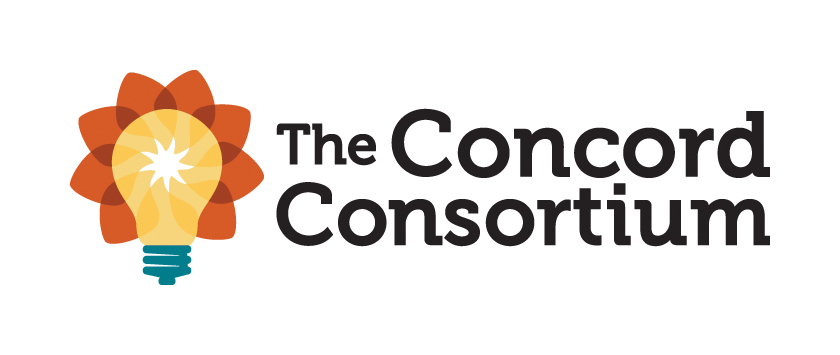HOT: Concord Consortium: SmartGraphs is a collection of graph-related activities to learn algebra.  Each includes interactivity to help with understanding.  Scaffolds and hints are provided to correct errors.  Standards are included.

Curriki Algebra 1 Aligned to the CCSS-M Standards is another algebra 1 offering designed for grade 9 learners with resources organized by Common Core math standards.  Per its description, "Both teacher facing and student facing assets are included and are of various forms, e.g. text, video, and animations."

Curriki Prealgebra Aligned to the CCSS-M Standards is designed for grade 8 learners with resources organized by Common Core math standards.  Per its description, "Both teacher facing and student facing assets are included and are of various forms, e.g. text, video, and animations."

Dave's Short Course on Complex Numbers

Get the Math is a multimedia project about algebra in the real world, which is for middle and high school learners.  Begin by viewing videos that show how professionals working in fashion, videogame design, music production, basketball, the restaurant business, and the special effects industry use algebraic thinking.  They present challenges connected to their jobs to two teams of teens.  "At that point viewers are encouraged to try the challenges themselves using interactive tools provided on the GET THE MATH website, before returning to the video to see the teams’ solutions.  Students can further explore the same algebra concepts through additional interactive challenges on the website" (About section).  The project is made possible by the Moody's Foundation THIRTEEN in association with WNET New York Public Media.

HOT!: Graspable Math is a free web-based tool to help students interactively explore and solve algebra equations by moving terms around on a screen--also a good tool for homework.  Several video tutorials are available to learn how to use mouse and touch gestures for algebra, such as the nature of the online "canvas" itself, the on-screen keyboard, simplifying expressions, working with the distributive and commutative properties, entering and solving equations, rewriting expressions, and more.  There are options to undo/redo, save, load, and share.  Teachers will value the additional materials, including activities for grades 6-12, and use of Graspable Math as a classroom presentation tool.  Graspable Math also has two game-based learning programs that help develop algebraic thinking: From Here to There! and From Here to There!: Elementary Edition.  The development of these resources was supported by the Institute of Education Sciences.

IXL Algebra 1 and IXL Algebra 2 problem sets address the skills that students learn in Algebra 1 and Algebra 2.  As students practice online and demonstrate mastery, the level of difficulty increases.  Skills are organized in categories.

Lumen Prealgebra is an interactive exploration of prealgebra topics.  The course is open-source and includes "whole numbers, algebraic notation, integers, fractions, decimals, percents, real numbers, multi-step linear equations, polynomials, geometry, and graphs."  Videos, problem sets, and online assessments are provided (About section).

MathBitsNotebook: Algebra 1 and Algebra 2-- Each of these sections includes a series of lesson and practice pages for high school Algebra. Materials cover all CCSS math standards and coordinate with SBAC, PARCC, and state assessments.  You'll also find two end-of-course interactive multiple-choice practice tests in each section related to CCSS Algebra 1 and CCSS Algebra 2.

Math 101: College Algebra is a free online course from the Study.com Academy.  It consists of video lessons and self-grading quizzes that prepare students to earn college credit in the U.S. These modular lessons, each about 10 minutes long, are taught by subject-matter experts and are animated and edited by a professional video-editing team.  Lessons have been reviewed by community college and university level instructors.  No log-in is required, so anyone studying introductory algebra can benefit from the videos.

Math Planet includes four courses: Pre-Algebra, Algebra 1, Algebra 2, and Geometry.  Each comes with multiple chapters divided into several lesson topics.  Under each lesson, you'll find theory, examples, and video lessons.  Math Planet also includes ACT and SAT practice questions.  Content is free.

Open Course Library from the Washington State Board for Community and Technical College features multiple courses and course materials, free to use under a Creative Commons license.  Descriptions are provided for each course:

• Elementary Algebra covers "solving and graphing linear equations, polynomials, factoring, and rational expressions."
• Intermediate Algebra covers "compound inequalities, systems of equations, radicals, rational equations and applications, and function notation."

OpenStax has open-source free online math textbooks, which are also peer-reviewed:

• PreAlgebra
• Elementary Algebra
• Intermediate Algebra
• College Algebra
• Algebra and TrigonometryPurplemath is a "not to miss" Algebra resource with lessons on preliminary, beginning, intermediate, advanced topics, and word problems.  An extensive list of Internet resources related to Algebra is also provided, including a link to online calculators.  This site, developed by E. Stapel of Western International University, is award winning.

Schmoop Pre-Algebra is online and free.  Content is arranged in six learning guides: basic operations, fractions and decimals, ratios and percentages, basic geometry, basic statistics and probability, and basic algebra.  There are succinct explanations, diagrams, interactive examples, practice problems, and real-world applications.  Also see the Algebra Introduction and its multiple study guides on algebra topics.

Science Friday: Explosion Math is a Common Core-aligned lesson in which students use linear equations (y=mx+b) to "to answer the age-old question of who would win between an Olympic sprinter, tortoise, car, you, and a volcano."  To find out, students calculate how much of a head start each would need to run away and escape from two different volcanoes that had erupted in 2018.  Brian Soash of Science Friday designed this lesson for students in grades 6-12.  It can be completed in 1-2 hours and includes all supporting materials and illustrative images for classroom use.

Study.com has online math courses in pre-algebra, algebra, geometry, statistics, precalculus, calculus, college math and more.  These feature video lessons from experienced educators, transcripts of those lessons, supplementary content, quizzes, and exams.  Learners can potentially earn college credit.

The Lost Function from Advanced Training & Learning Technology provides pre-algebra game-based learning using content aligned to the Common Core standards, grades 6-8.  The curriculum is comprehensive covering topics in

1. Whole Numbers
2. Integers
3. Fractions & Decimals
4. Exponents & Roots
5. Rates, Ratios, & Proportions
6. Equations
7. Inequalities
8. Graphing
9. Probability & Statistics
10. Geometry

An "in-game virtual math tutor monitors progress and teaches concepts when you need it most."  The storyline involves a town that has lost its ability to do math and a character Pi who is the only hope to help the people re-learn their skills.  The pre-algebra bundle comes with a price of about \$25, with school volume discounts available.

Mathmistakes.info: Common Algebra Mistakes is a list of common errors found in algebra listed by topic and what the correction should be.  These are from actual student errors.  The site also contains common trigonometry and calculus mistakes.

Virtual Math Lab from West Texas A&M University concentrates on Beginning Algebra, Intermediate Algebra, and College Algebra.  Each course has complete online tutorials (many with video explanations), practice problems with answers, and resources for extra help.  Anyone can use these.

Why U contains animated videos in pre-algebra and algebra.  "Rather than focusing on problem solving, the objective is to give insight into the concepts on which the rules of mathematics are based."  Beginning with a humorous look at the Dawn of Numbers, the pre-algebra tutorial videos (over 30 of them) lay the foundation for high school and college algebra.  The algebra tutorial videos (over 70 of them) explain not only the how, but why the rules of algebra work.  Videos were written by Steve Goldman and animated by Mark Rodriquez.  The animations are outstanding.

Wolfram for Education has some free resources for teaching math.  For example, see Using Wolfram|Alpha in the Classroom.  This latter is useful for teaching topics in Algebra and getting step-by-step solutions to problems, including graphing.  Lesson plans are also available.

WOWmath features hundreds of videos developed by math teacher, Brad Robb, for algebra 1 and algebra 2.  They correlate with 2008 Holt - Algebra 1 California Edition; and 2008 Prentice Hall - Algebra 2 California Edition.

### Algebra on your mobile devices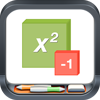Algebra Tiles from Brainingcamp
Price: \$1.99
Target: Grades 6-8 (Note: learners studying algebra 1 will also benefit.)
Info: These algebra tiles can be used to explore 1) adding, subtracting, multiplying, and dividing integers, 2) modeling and simplifying expressions, 3) substitution, 4) adding and subtracting polynomials, 5) solving equations and systems of equations, 6) distribution, 7) adding, subtracting, multiplying and dividing polynomials, 8) factoring, and 9) completing the square (Product description).  Brainingcamp also has other apps for iPads.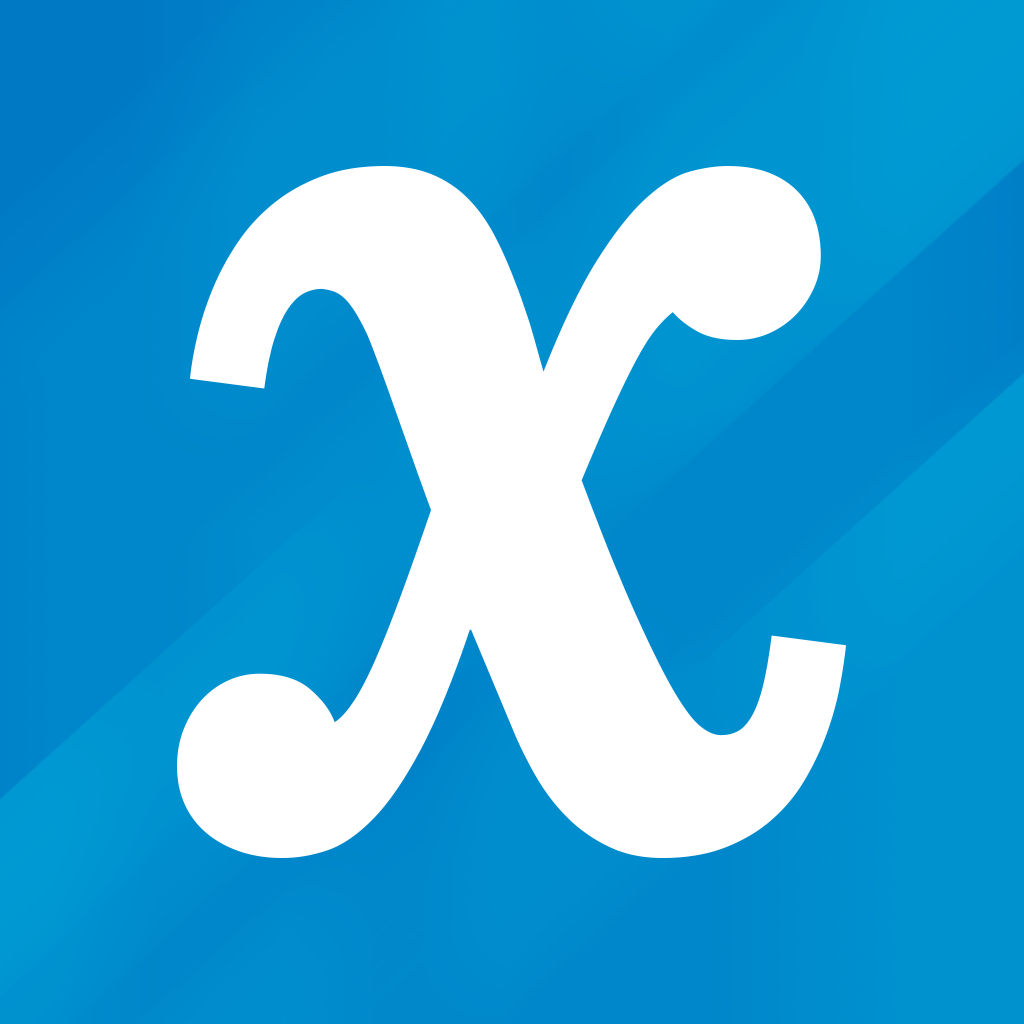Algebra Touch: All Ages by Regular Berry Software LLC for iPhone, iPod Touch, and iPad (free with in-app purchases) offers users touch-based techniques to help them refresh algebra skills.  It covers the following: "Simplification, Like Terms, Commutativity, Order of Operations, Factorization, Prime Numbers, Elimination, Isolation, Variables, Basic Equations, Distribution, Factoring Out, Exponent Product Rule, and Exponent Quotient Rule."  See the video at http://www.regularberry.com/Find X Algebra Pro by Flooki is an equation solver designed for iPad.  It is available for \$1.99.  Per its description, "Find x of an algebraic equation in less than a second. You have both sides of an equation where you can enter your algebraic terms. After entering the algebraic terms this app shows every step how to transform your complicated equation into a very simple one. In the end a numeric algorithm solves the simplified equation and provides the solution for x. It finds x (or the zeros of a function)."  There are no ads.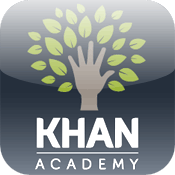Khan Academy app is free and compatible with iPhone, iPad, and iPod touch.  You can learn just about anything.  Multiple subjects are included.  "Learn using videos, interactive exercises, and in-depth articles in math (arithmetic, pre-algebra, algebra, geometry, trigonometry, statistics, calculus, linear algebra)."McGraw Hill AR by McGraw Hill and Verizon
Platform: Apple or Android tablet or smartphone
Price: Free
Info: This is an augmented reality app with standards-aligned lessons featuring algebra readiness activities and 3D geometry concepts.  For example, you'll find lessons on cross sections, nets, rotations, slope, solving equations, function growth, parallel and skew lines, transformations in the coordinate plane, Pythagorean theorem in 3D, brain teasers, with more to come.  Lessons are available at Verizon Innovative Learning HQ.Socratic--Homework helper by Google is a free app compatible with iPhone, iPad, iPod Touch, and Android.  It's designed primarily for high school and university learners.  You can take a photo of your homework question, typed or handwritten, and get explanations, videos, and step-by-step help.  The app also includes voice recognition.  When you pose a question, "the app will find the best online resources for you to learn the concepts."  Supports Math, Science, Social Studies, Literature, and more.  For example, math supports algebra, geometry, trigonometry.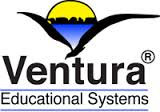Ventura Educational Systems has a variety of IOS apps for iPad and iPhone.  Prices depend on the app.  For example, apps for algebra:

• Algebra Concepts
• Algebra Balance
• Algebra Tiles
• Algebra 1 Quick Reference
• Simultaneous Equations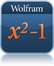Wolfram Algebra Course Assistant is available for a couple of dollars. Look for the title on your preferred device: iOS, Android, or Windows.  See it on the Web.  Per the description: This app covers topics in Algebra I, Algebra II, and College Algebra.  It can:

• Evaluate any numeric expression or substitute a value for a variable.
• Simplify fractions, square roots, or any other expression.
• Solve a simple equation or a system of equations for specific variables.
• Plot basic, parametric, or polar plots of the function(s) of your choice.
• Expand any polynomial. Factor numeric expressions, polynomials, and symbolic expressions.
• Divide any two expressions.
• Find the partial fraction decomposition of rational expressions.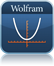Wolfram Pre-Algebra Course Assistant assists with many topics covered in pre-algebra courses, including solving equations, simplifying expressions, and plotting equations on the coordinate plane.  Look for the title on your preferred device: iOS, Android, or Windows.  See it on the Web.

### Do you need practice for End-of-Course Exams?Several state departments of education post released test items from prior end-of-course exams.  See our Standardized Test Preparation page for a link to your state.

#### Do you need Common Core Performance Tasks for Math?

The Mathematics Assessment Project has both summative tests or tasks and formative assessment lessons.  Tasks available are for high school and middle school, also linked to specific Common Core standards.  Tasks are grouped into novice, apprentice, and expert levels and "variously ask students to use their mathematics in routine or non-routine situations to design, plan, estimate, evaluate and recommend, review and critique, investigate, re-present information, explain, define concepts, and show their skills in routine technical exercises."

## Geometry

### HOT NEWS!Mathematicians Excited About New 13-Sided Shape Called 'the Hat' (2023).

In March 2023, Kevin Hurler at gizmodo.com reported that "Researchers [mathematician David Smith and colleagues] identified a shape that was previously only theoretical: a 13-sided configuration called “the hat” that can tile a surface without repeating. The hat is what’s known as an aperiodic monotile, which means that a single shape can tile a surface without any translational symmetry, or without its pattern ever repeating." (para. 1-2)

So what does it look like?

"The 13-sided hat is a polykite shape, consisting of eight kites [highlighted in gray in the image] connected at their edges."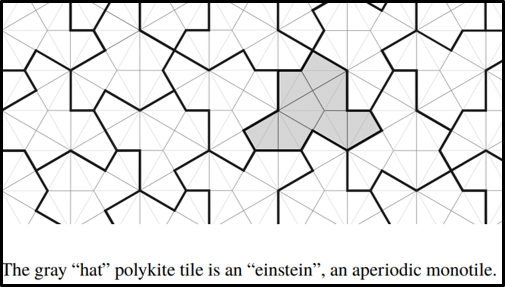Image source: David Smith et al.

Smith, D., Myers, J., Kaplan, C., & Goodman-Strauss, C. (2023, March 20). An aperiodic monotile [Preprint]. https://arxiv.org/pdf/2303.10798.pdf

### What is Geometry?Although they may be in your geometry class, consider that student levels of geometric thinking might not be sufficient to engage in formal proofs.

View the Mind Map of the van Hiele Model of Geometric Thought at GoGeometry.com.  The Van Hiele model asserts that learners develop geometric thought sequentially through five levels of understanding.  Numbered as levels 0-4, they are visualization, analysis, informal deduction, deduction, and rigor.  Hopefully, students have progressed to the level of informal deduction by the end of grade 8.  Read more on The van Hiele Levels of Geometric Understanding by Marquerite Mason for further implications for teaching.

Students might also benefit from the following Dr. Math books (2004) from The Math Forum.

Educators might be interested in the Ohio Geometry Blueprint, which is helpful for planning curriculum and guiding instruction.  It includes the standards for congurence and proof, similarity and trigonometry, circles, and probability typical for a geometry course.  These standards are eligible to appear in the state year-end standardized test for geometry.

#### Byrne's Euclid

Euclid's Elements has been the foundation for hundreds of editions of geometry textbooks since he first created his 13 books dating back to around 300 BC.  In 1847 Oliver Byrne's edition of the first six books became noteworthy owing to his use of multiple colors in diagrams to help explain proofs and make geometry easier to understand.  In recent times, web designer and artist Nicholas Rougeux reproduced Byrne's Euclid as an interactive website staying true to its color scheme, graphics, and typography.  Rougeux's clickable shapes and links to cross-references in the text add value to exploring his project, which was launched in December 2018.  You can read how he did it in his blog post, Making of Byrne's Euclid.Curriki Geometry Aligned to the CCSS-M Standards "is a collection of resources for a high school course in Geometry. The resources are organized by Common Core State Standards for Mathematics and reflect a ninth or tenth grade course. Both teacher facing and student facing assets are included and are of various forms, e.g. text, video, and animations" (Content section description).

Curriki: Project Based Geometry is a free curriculum that takes a project-based approach for learning.  There are several Common Core State Standards aligned projects, each of which focuses on at least two of the eight mathematical practice standards and includes a rubric for assessing mathematical practices.  Teachers can select to use all or just some of the projects.  Projects can be taught in any order and include technology and Web 2.0 resources "such as videos, documents, web pages, and dynamic geometry constructions, quizzes and exam suggestions for assessment, and other tools related to the project."  You'll find:

1. Selling Geometry
2. Designing a Winner
4. TED Talk: House of the Future
5. The Art of Triangles
6. How Random is My Life?

Floor Planner can be used with learners in grades 6-12 to interactively design floor plans in 2-D and 3-D using point-and-click, drag-and-drop tools, and then share them with others.  Consider scale drawings for studying geometric shapes, spatial relationships, and ratio/proportions, for example. Students can also show their creativity, and move elements of their design (e.g. furnishings) around as often as they like.  Its free for personal use.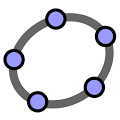GeoGebra is free, open-source, dynamic mathematics software that joins geometry, algebra, tables, graphing, statistics, and calculus.  You can do constructions with points, vectors, segments, lines, conic sections as well as functions and change them dynamically afterwards.  You can enter equations and coordinates directly. GeoGebra has the ability to deal with variables for numbers, vectors and points, finds derivatives and integrals of functions and offers commands like Root or Extremum.  It received several international awards, including the European and German educational software awards. In Dynamic Mathematics with GeoGebra, authors Markus Hohenwarter, principle developer of the software, and Judith Preiner (2007) said, "You can use GeoGebra both as a teaching tool and to create interactive web pages for students from middle school up to college level. Specifically designed for educational purposes, GeoGebra can help you to foster experimental, problem-oriented and discovery learning of mathematics" (Abstract section).  They illustrate the basic ideas of the software and some of its versatile possibilities by discussing several interactive examples.  Additional resources for using GeoGebra include:

Geometry 3-D Shapes is an interactive from Annenberg Learner intended for grades 6-8, although other grades can benefit.  Students can interactively learn about three-dimensional shapes, calculate surface area and volume, and discover some of the mathematical properties of shapes. It comes with a glossary, tutorials, and an interactive test.

Geometry Applet Gallery from Saltire Software contains some excellent applets, which are easy to use and suitable for illustrating several theorems presented in a high school or post-secondary geometry course.  Basic Geometry relates to angles in parallel lines, circular configurations, Pythagorean triples and incircles. Students can drag points to explore concepts.  Four triangle calculators are presented.  By entering SSS, SAS, or ASA, students can see the unique triangle that is formed, but if SSA is entered the resulting triangle is not unique (two are possible) or no triangle is possibly formed with given information.  Some advanced geometrical configurations are explored.  Saltire's work has been assisted with a number of National Science Foundation grants.

Geometry Playground is a free Java applet from the Mathematical Association of America Mathematical Sciences Digital Library Loci Resources, which enables "ruler and compass" constructions in "Euclidean, Spherical, Projective, Hyperbolic, Manhattan and Conical geometries" (Description section).  Potential classroom activities are included.

HOT!: GoGeometry from the Land of the Incas, by Antonio Gutierrez, is an award-winning site that is intended to raise the interest of students in Euclidean geometry. The site includes geometry theorems and problems, proofs, online interactive geometry puzzles, drawings, infographics, mobile apps, mindmaps, and more.  There's a section of problems developed using GeoGebra with step-by-step instructions on how to use each interactive.  Users can view and post solutions to the geometry problems.  Best used with high school students and above.

IXL Geometry problem sets address the skills that students learn in Geometry.  As students practice online and demonstrate mastery, the level of difficulty increases.  Skills are organized in categories.

MathBitsNotebook: Geometry "is a series of lesson and practice pages for students studying high school Geometry. These materials cover ALL standards stated in the Common Core State Standards for Mathematics (Appendix A, traditional pathway for Geometry), and coordinate with both SBAC and PARCC assessments" (Website description).

Math Open Reference includes a free geometry textbook with an introduction to trigonometry for high school learners by John Page.  There are topics in plane geometry, coordinate geometry, and solid geometry (e.g., cylinders, cubes). Its focus is on using interactive materials and animations to develop concepts.  Sections on constructions are excellent.

Math Planet includes four courses: Pre-Algebra, Algebra 1, Algebra 2, and Geometry.  Each comes with multiple chapters divided into several lesson topics.  Under each lesson, you'll find theory, examples, and video lessons.

Poly is a shareware program for exploring and constructing polyhedra.  You can manipulate solids on the computer and also print out the net of the polyhedra so that you can create the physical 3-D model.  Poly is downloaded from Pedagoguery Software.

### Geometry on your mobile devices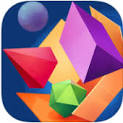Arloon Geometry (\$2.99) for Android and iOS devices is for students ages 11 and up.  It "features 3D models with Augmented Reality for most geometric shapes" and enables users to "View geometric shapes from all angles and see their sides unfold into flat figures. Geometry combines conceptual content (definitions and characteristics) with procedural content (applying formulae and calculus)."  Practice exercises are included: "guess the geometric shape, confirm characteristics and calculate area and volume."  It's available in English and Spanish.  The app is also posted on edshelf.com, which includes other augmented reality math apps.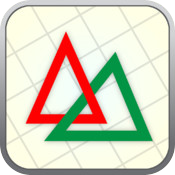Ezy Triangle by KGiants is for iPad and iPhone.  This free app for high school learners and above is designed to solve triangles given any 3 out of 6 quantities (sides and angles).  It can handle an ambiguous case of multiple solutions and allows interacting with the triangle.  It displays side lengths of the triangle, has auto-saving of the triangle and comes with a list of triangle-related formulas.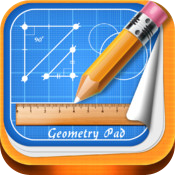Geometry Pad by Bytes Arithmetic LLC is a free dynamic geometry iPad app.  "With the Geometry Pad you can create fundamental geometric shapes, explore and change their properties and calculate metrics. The shapes are displayed on a scrollable and zoomable workbook with a rectangular coordinate system" (Description section). Multiple tools are built into the application: move & scale, point, line, midpoint; parallel, perpendicular, tangent lines; angle, triangle, triangle lines, quadrilaterals, circles, radii, chords, polygons, arcs, sectors, conic sections, measurements and transformations tools, manual inputs, calculator functions, and more.iCrosss by Oleh Yudin helps users learn solid geometry.  Per the app description, it comes with about 50 polyhedra, including " regular polyhedra (Platonic solids), Pyramids, Prisms, Anti-prisms, Archimedean solids and duals."  You can rotate the 3D solid in any direction, add a plane to visualize a cross-section of it, get information about the solid ("short description, number of faces, vertices, edges, web-link for more information and useful formulas"), separate it into parts in 3D cut mode, print and email it.  It's available for iPad, iPhone, and Android devices for \$.99.Khan Academy app is free and compatible with iPhone, iPad, and iPod touch.  You can learn just about anything.  Multiple subjects are included.  "Learn using videos, interactive exercises, and in-depth articles in math (arithmetic, pre-algebra, algebra, geometry, trigonometry, statistics, calculus, linear algebra)."McGraw Hill AR by McGraw Hill and Verizon
Platform: Apple or Android tablet or smartphone
Price: Free
Info: This is an augmented reality app with standards-aligned lessons featuring algebra readiness activities and 3D geometry concepts.  For example, you'll find lessons on cross sections, nets, rotations, slope, solving equations, function growth, parallel and skew lines, transformations in the coordinate plane, Pythagorean theorem in 3D, brain teasers, with more to come.  Lessons are available at Verizon Innovative Learning HQ.Ventura Educational Systems has a variety of IOS apps for iPad and iPhone.  Prices depend on the app.  For example, apps for geometry:

• Geometry Concepts
• Coordinate Geometry
• Geometry Quick Reference
• Graph Power
• Geometric Transformations
• Circular Geoboard

## Trigonometry and Precalculus

### What is trigonometry?

Taken from the 1994 book The Words of Mathematics by S. Schwartzman and posted at cut-the-knot.org

trigonometry (noun), trigonometric (adjective): the first part of the word is from Greek trigon "triangle". The second part of trigonometry is from Greek metron "a measure." The Indo-European root is probably me- "to measure." Trigonometry is literally the measuring (of angles and sides) of triangles. Historically speaking, the triangular approach to trigonometry is ancient, wheres the circular approach now taught in our schools is relatively recent.

### Are you ready for trigonometry and precalculus?Students: The CalcWorkshop has posted an online PreCalculus Diagnostic Test with answers.  A series of video solutions is also included.

You can also take the Pre-Calculus and Trigonometry Readiness Test provided by HSLDA Online Academy.  The test is helpful in making a decision regardless of where you attend school.Dave's Short Trig Course, by Dave Joyce of Clark University in Massachusetts, contains explanations, practice problems and answers.  Best of all is that images are virtual manipulatives developed in Java so that learners can explore the properties presented.  A brief background of the Pythagorean Theorem and similar triangles is included.  See also Dave's Short Course on Complex Numbers.

Exploring Precalculus, by Dr. William Mueller, provides an introduction to precalculus using Mathcad or StudyWorks as a calculation and visualization tool. Many interactive examples let you explore the concepts and practice the skills necessary for understanding calculus.  Central themes (functions, rates of change, and accumulation) are developed intuitively, from several perspectives (algebraic, numeric, and graphic).  Materials suitable for use in grades 9-12, and post-secondary.

IXL Precalculus problem sets address the skills that students learn in Precalculus.  As students practice online and demonstrate mastery, the level of difficulty increases.  Skills are organized in categories.

Law of Sines and Law of Cosines are two applets that allow you to input sides and angles per each law and then each applet calculates the missing sides and angles in a triangle.  Some practical applications of each law are also included.  These are hosted by the Department of Physics and Astronomy at Georgia State University.

Open Course Library from the Washington State Board for Community and Technical College features multiple courses and course materials, free to use under a Creative Commons license.  Descriptions are per each course.

• Precalculus 1 covers "families of functions, their properties, graphs and applications.  These functions include: polynomial, rational, exponential, logarithmic functions and combinations of these. [Learners] will solve related equations and inequalities and conduct data analysis, introductory mathematical modeling and develop competency with a graphing calculator."
• Precalculus II addresses "families of trigonometric functions, their inverses, properties, graphs, and applications. Additionally, [learners] study trigonometric equations and identities, the laws of sines and cosines, polar coordinates and graphs, parametric equations and elementary vector operations."

OpenStax has open-source free online math textbooks, which are also peer-reviewed:

• Algebra and Trigonometry
• Precalculus

If you are using the OpenStax Algebra and Trigonometry or Precalculus, students will benefit from the resources from OpenStax available in the Möbius Content Packs posted at DigitalEd.com.  The resources are designed to help them learn and practice concepts.  Multiple question types are available.

The Math Page: Trigonometry addresses 20 trig topics and provides examples and problems.  The section for Precalculus includes over 25 topics.  The site is by Lawrence Spector.

Study.com has online math courses in pre-algebra, algebra, geometry, statistics, precalculus, calculus, college math and more.  These feature video lessons from experienced educators, transcripts of those lessons, supplementary content, quizzes, and exams.  Learners can potentially earn college credit.

### Trig and Precalculus on your mobile devices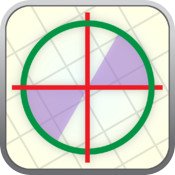Ezy Trigonometry by KGiants is for iPad and iPhone.  This free app for high school learners, or others learning trigonometry, features an interactive unit circle to explore relationships between angles and trig ratios.  It comes with an exact trig ratios table, a handbook of trig formulas, and settings for radians/degrees, related angles, and angle snapping.Khan Academy app is free and compatible with iPhone, iPad, and iPod touch.  You can learn just about anything.  Multiple subjects are included.  "Learn using videos, interactive exercises, and in-depth articles in math (arithmetic, pre-algebra, algebra, geometry, trigonometry, statistics, calculus, linear algebra)."Wolfram Precalculus Course Assistant is available for iOS, Android, and Windows. See it on the Web   It's priced for a couple of dollars and covers topics in precalculus and trigonometry.  It can:

• Evaluate any numeric expression or substitute a value for a variable
• Solve a single equation or a system of equations
• Plot functions on the x-y plane or draw a parametric or polar plot
• Determine the sine, cosine, and tangent of a specific angle in a right triangle
• Simplify, expand, or factor trigonometric functions
• Find the partial fraction decomposition of an expression
• Calculate the dot product, cross product, and magnitude of two vectors
• Identify the mean, median, mode, and standard deviation of a set of data
• Calculate permutations and combinations (Description section).

### Tired of using 3, 4, 5 right triangles?

Generate your own sides of the right triangle.  Using positive integers s and t with s< t, calculate legs x and y and hypotenuse z as follows:Get a quick list of Pythagorean Triples using a simulation from the University of Illinois Office of Mathematics, Science, and Technology Education.

Pythagorean Triples Generator at Desmos is quick.  The generator is inspired by its explanation on Constructing Pythagorean Triples at https://www.mathsisfun.com/numbers/pythagorean-triples.html, which also contains a list of such triples.

## Calculus

### Do you know what calculus is and where it is used?In What Exactly Is Calculus And How Do We Use It In Everyday Life? posted at ScienceABC, Venkatesh Vaidyanathan (2022, July 9) delved into the history of calculus, the nature of differential and integral calculus and how they are connected.  He provided specific examples of its use in aerospace engineering and medicine and mentioned that calculus is used in "diverse fields such as microeconomics, astrophysics, the stock market and radioactive decay.  Anything that involves studying the rate of change and finding an optimal solution..."

### Are you ready for calculus?

Students: The University of California at San Diego has posted an online Calculus Readiness Test with answers.  Many of the problems are challenging, but don't give up.

See resources, including released questions from prior exams, at the College Board AP Central for both the Calculus AB and Calculus BC exam levels.  Also see the AP Calculator Policy.  Graphing calculators are required for the AP Calculus exams and a list is provided on those that are acceptable.Active Learning Materials for Calculus includes resources for precalculus, and first, second, and third semesters of calculus.  These are provided by the University of Colorado at Boulder.

AP Calculus is a free self-paced online course from Davidson Next, which addresses the "most difficult topics in your AP Calculus AB & Calculus BC courses."  Per the course description, "tricky topics are broken up into bite-sized pieces—with short instructional videos, interactive graphs, and practice problems written by many of the same people who write and grade your AP® Calculus exams."  It can be used in a blended classroom or as supplementary help and exam review for students.  Topics include limits, definition of derivative, chain rule, implicit differentiation, L'Hospital's Rule, Riemann sums, functions defined by definite integrals, modeling and solving differential equations, rectilinear motion, parametric equations, introduction to series, series convergence, and series manipulation.

Applied Calculus: Everything! by Stefan Waner is a complete text beginning with a precalculus review, topics in finite mathematics, then study of differentiation and integration with applications.  There are online tutorials, tools for exploration, review exercises, quizzes, and more.

Calculus, posted at Curriki.org, is described as "a very full course of Calculus videos, including such topics as Limits, derivatives, definite and indefinite integrals, minima and maxima, word problems, etc."

Calculus Online Textbook by Gilbert Strang is posted at MIT OpenCourseware.  The book "is a useful resource for educators and self-learners alike. It is well organized, covers single variable and multivariable calculus in depth, and is rich with applications. There is also an online Instructor's Manual and a student Study Guide."  Dr. Strang also has a series of videos "to show ways in which calculus is important in our lives."  They include "real-life examples to illustrate the concepts."

Calculus-help.com by M. Kelley has been recognized by The National Council of Teachers of Mathematics and The College Board.  Don't miss the section of Tutorials for the Calculus Phobe, an ongoing project to present topics from this subject in an easily understood audio-visual format.  Flash animation is incorporated.  Topics include limits, continuity, differentiation, with more to come.

Calculus Revisited, posted at the MIT OpenCourseWare initiative, features a series of videos and related resources on topics covered in an introductory calculus course.

Capsules for One-Variable Calculus is brought to you by the Mathematical Association of America within its digital library, MathDL.  It is a compendium of learning capsules in different areas appropriate for anyone teaching one-variable calculus.  Among topics are general tools, continuity, limits, differentiation, the Fundamental Theorem, antidifferentiation, integration, differential equations for calculus, function approximation, theoretical issues, and more.  Content has been vetted by leading experts in the field of mathematics and math education.

Derivative Calculator is free and finds derivatives of functions.  "The Derivative Calculator supports computing first, second, …, fifth derivatives as well as differentiating functions with many variables (partial derivatives), implicit differentiation and calculating roots/zeros. You can also check your answers! Interactive graphs/plots help visualize and better understand the functions" (About section).

Elementary Calculus: An Infinitesimal Approach, On-line Edition, copyright 2000 and revised 2022 by H. Jerome Keisler, is a freely-available calculus text from the author.  You can download the whole book or selected chapters.

GeoGebra: Calculus Applets by Marc Renault of Shippenburg University includes his excellent collection of applets on limits, differentiation, applications of the derivative, and integrals.  Applets are written using HTML5.

GeoGebra: Calculus Applets by Tim Brzezinski is a collection of applets that relate to limits and continuity, derivatives, integrals, differential equations, polar coordinates, parametric equations, sequences and series, multivariable calculus, and more.

GeoGebra: Calculus I, II, and III lessons and applets by Tuyetdong Phan-Yamada, a math department lecturer at California State University, Los Angeles.  Phan-Yamada also has lessons and applets for discrete math, differential equations, statistics, finite math, algebra (linear, beginning, intermediate, college) and geometry.

Integral Calculator is free and finds integrals and antiderivatives of functions.  It shows you the full step by step integration.  "All common integration techniques and even special functions are supported. The Integral Calculator supports definite and indefinite integrals (antiderivatives) as well as integrating functions with many variables. You can also check your answers! Interactive graphs/plots help visualize and better understand the functions" (About section).

IXL Calculus problem sets address the skills that students learn in Calculus.  As students practice online and demonstrate mastery, the level of difficulty increases.  Skills are organized in categories.

Math 104: Calculus is an online course from the Study.com Academy.  It consists of over 100 video lessons and self-grading quizzes that prepare students to earn college credit in the U.S. These modular lessons, each about 10 minutes long, are taught by subject-matter experts and are animated and edited by a professional video-editing team.  Lessons have been reviewed by community college and university level instructors.

Math Insight includes numerous pages with content relevant for calculus, including applets.  The general index will provide a helpful look at topics included.

Math Vault: Calculus contains a series of essays with explanations on a variety of calculus topics.  Examples include, but are not limited to the following:

• The Algebra of Infinite Limits — and the Behaviors of Polynomials at the Infinities
• Chain Rule for Derivative -- The Theory
• Exponent Rule for Derivative: Theory & Applications
• Integration Series: The Overshooting Method
• Derivative of Inverse Functions -- Theory & Applications

Open Course Library from the Washington State Board for Community and Technical College features multiple courses and course materials, free to use under a Creative Commons license.  Descriptions are provided for each course: Calculus I, Calculus II, Calculus III.

Open Culture includes free math courses from top universities.  You can download these audio and video courses ranging from algebra to calculus and statistics.  Other content areas are included.

OpenStax open-source free online math textbooks, which are also peer-reviewed:

• Calculus Volume 1
• Calculus Volume 2
• Calculus Volume 3

If you are using the OpenStax Calculus volumes, students will benefit from the calculus resources from OpenStax available in the Möbius Content Packs posted at DigitalEd.com.  The resources are designed to help them learn and practice concepts.  Multiple question types are available.

Study.com has online math courses in pre-algebra, algebra, geometry, statistics, precalculus, calculus, college math and more.  These feature video lessons from experienced educators, transcripts of those lessons, supplementary content, quizzes, and exams.  Learners can potentially earn college credit.  Study.com also has a specific collection of video lessons and practice tests for AP Calculus AB & BC: Exam Prep.

Teaching Calculus by Lin McMullin is a blog for high school teachers and students, especially Advanced Placement Calculus teachers and students.  You'll find comments, suggestions, hints, and observations on the topics for the course, and a video collection.  Of particular value is the Blog Guide section, which includes 10 units with calculus topics in an order that they are typically taught.

Video Calculus is a collection of over 50 lectures provided in two video formats that were developed by Selwyn Hollis of the Mathematics Department at the University of Houston.  Videos cover a full range of topics for calculus I and calculus II.

Walter Fendt's HTML5 Applets on Mathematics address arithmetic, plane geometry, trigonometry (visual demonstration of graphs of sine, cosine and tangent of an angle), vector calculation, analysis (e.g. app to introduce the derivative, 1st and 2nd derivatives), and complex numbers (arithmetic operations).  These are very engaging and illustrative of concepts.

Why U contains animated videos in pre-algebra and algebra, topology, and infinite series.  The infinite series animation "makes complex concepts such as summation notation, infinitesimals, and convergence easily comprehensible."  Videos were written by Steve Goldman and animated by Mark Rodriquez.  The animation is outstanding.

Wolfram for Education has some free resources for teaching math.  For example, see Using Wolfram|Alpha: Examples for Calculus and Analysis.  This latter is useful for teaching topics in Calculus and getting step-by-step solutions to problems: derivatives, integrals, graphing, and so on.  Free unlimited practice problems are also available.

Wolfram Alpha online calculus calculators:

WOWmath features hundreds of videos developed by math teacher, Brad Robb, for AP calculus (AB level).  They correlate with the 8th Edition Houghton Mifflin - Calculus of a Single Variable.

### Calculus on your mobile devices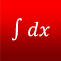Calculus Pro by Richard Ssenyonjo is compatible with iPhone, iPod Touch, and iPad.  It's free and includes in-app purchases.  It features topics in differential and integral calculus and differential equations.  It is suitable for beginners to advanced learners with multiple hints provided.Khan Academy app is free and compatible with iPhone, iPad, and iPod touch.  You can learn just about anything.  Multiple subjects are included.  "Learn using videos, interactive exercises, and in-depth articles in math (arithmetic, pre-algebra, algebra, geometry, trigonometry, statistics, calculus, linear algebra)."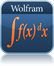Wolfram Calculus Course Assistant is available for a couple of dollars.  It covers topics applicable to Calculus, AP Calculus AB, AP Calculus BC, Calculus I, and Calculus II.  Look for the title on your preferred device: iOS, Adroid, or Windows.  See it on the Web.

### How Big is Infinity?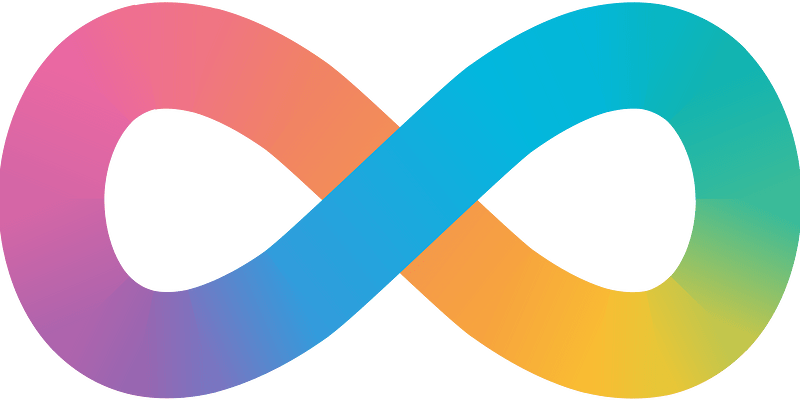Some learners first encounter a formal study of infinity when they take their first course in calculus.  However, you don't have to wait to take calculus to get a sense of the size of infinity and to appreciate that there are some unanswered questions in mathematics.  View the video at TED-Ed in which educator Dennis Wildfogel used fundamentals of set theory and basic knowledge of real numbers to explore How Big is Infinity?  To stimulate conversation, there's also a quiz, questions to think about and to dig deeper.  This lesson can also be used in a flipped classroom.

In How Many is a Googol? posted at Wonderopolis.org, students can get a better appreciation of the difference between a really large number and infinity.  Even students just learning about powers of 10 will benefit from this.

Also at Wonderopolis.org is What is Infinity? Infinity is more than just a really large number.  There can be different sizes of infinity!  Really.

#### More Supplementary VideosDennis Wildfogel also has a series of videos on Making Sense of Calculus.  Several deal with the visualizing the derivative of terms such as x2, x3, x-1, x-2, x1/2, x1/3, x-1/2, x2/3, x3/2, then the Product Rule, and more to come.At 3Blue1Brown, a YouTube channel, author Grant Sanderson provides "some combination of math and entertainment, depending on your disposition. The goal is for explanations to be driven by animations and for difficult problems to be made simple with changes in perspective" (About section).  Calculus students will benefit from his playlist on topics such as the Essence of Calculus, the Paradox of the Derivative, Derivative Formulas through Geometry, Visualizing the Chain Rule and Product Rule, Derivatives of Exponentials, Implicit Differentiation, Limits, Integration and the Fundamental Theorem of Calculus.  Other playlists are available.

## Statistics and Probability

### What do we mean by Data and Probability?### What is Statistics?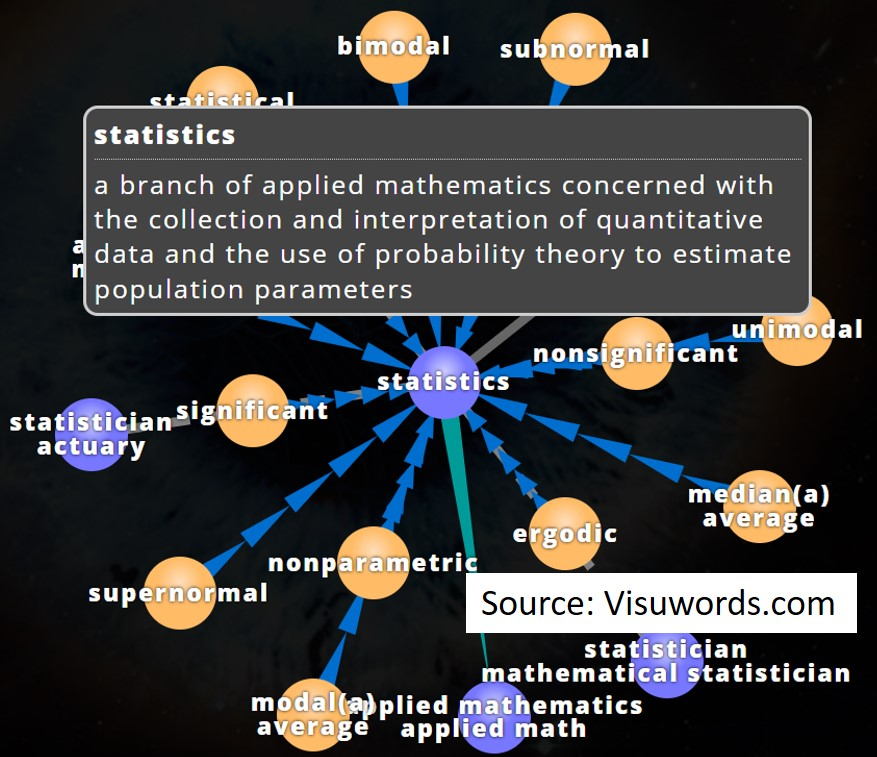AP Statistics Resources from JMP software by SAS.  Per the website: "This collection of lab activities provides step-by-step instructions and guidance on the exploration and application of statistical methods covered in AP Statistics courses. These can be used as in-class activities or homework assignments."  Learn about regression, hypothesis testing, confidence intervals, probability and sampling distributions, exploring and describing data, and more.  There's also a learning library with tutorials and videos.

ChartGizmo is free online software that can be used to create several kinds of graphs: Pie, Bar, Line, Ring, 3D Pie, 3D Bar, 3D Line, Candle, Scatterplot, TimeSeries.  A manual is available; chart settings can be customized. Several data sources are supported: static data, historical data, dynamic data from JavaScript code, dynamic data from html table on the page. Results can also be displayed on your own web pages.

HOT!: Crash Course Statistics is series of videos posted on YouTube that takes a closer look at how statistics play a significant role in everyday life "from batting averages and insurance rates to weather forecasting and smart assistants" and more.  The first of those was posted in January 2018.  You'll find episodes involving topics such as mathematical thinking, measures of central tendency and spread, data visualization, correlation, controlled experiments, sampling methods and bias in surveys.  This is a great motivational series and a math course, as well.

Data4Kids is a project from the Urban Institute and their partners.  It contains "data stories" that educators can use to teach about data, data science, and data visualization concepts to learners in virtual (or in-person) classrooms.  "Each story is a starter kit for educators at different levels: grades 3–5 (band 1), grades 6–8 (band 2), and grades 9–12 (band 3)."  Each includes four items: an instructor guide, data, data dictionary, teacher slides, and a zip file with those four items.  Story titles include City Health Equity, Evictions, Food Insecurity, Imagery Analysis, and National Parks.  Additional data resources are available.

Data Games from KCP Technologies are free online games suitable for learners in grades 6-12.  Each is linked to Common Core Standards for Math.  Lessons are designed to help learners to "analyze data, interpret and create linear and quadratic models, use proportional reasoning, and understand probability."  Student and teacher videos, worksheets, and teacher facilitation guidelines for classroom use are provided (For Teachers section).  Data can be saved.

DIG Stats is an excellent resource for integrating statistics and data visualization into mathematics and science courses in grades 9-14. Modules with student activities cover concepts from descriptive statistics, inferential statistics, two dimensional graphical analysis and three dimensional data visualization.  Using Microsoft Excel and graphing calculators, students analyze real world data sets from a variety of scientific disciplines.  Data were derived from WWW databases, university and government researchers, and student projects.  Guides, quizzes, and answers are provided.  This site is associated with the Central Virginia Governor's School for Science and Technology.

GeoGebra: Statistics Resources for High School includes interactives and simulations for statistics and probability, authored by the GeoGebra Team.

Good Calculators: Mathematics Statistics and Analysis Calculators.

Meta-Calculator: With the Statistics Calculator you can find standard deviations, correlation coefficients, do regression analysis, and perform t-tests.

Online Statistics Calculators: There are five calculators available for measures of central tendency and dispersion, box and whisker plots, linear regression, correlation coefficients, and scatter-plots.

Online Statistics Education: An Interactive Multimedia Course of Study is a free online textbook by David Lane, project leader at Rice University, and his co-authors.  Chapters also contain videos, simulations, and case studies.  For example, there are simulations with instructor notes for sampling, measurement, box plots, summarizing distributions, describing bivariate data, probability, normal and sampling distributions, confidence intervals, testing means, power, linear fits, ANOVA, and chi-square.  Educators who use the book can request additional materials, including an instructor guide and PowerPoint slides.

Open Course Library from the Washington State Board for Community and Technical College features multiple courses and course materials, free to use under a Creative Commons license.  Per its description, Introduction to Statistics introduces "some of the more common tools for analyzing data of several types. ...The main goal of the course is to highlight the general assumptions and methods that underlie all statistical analysis. The purpose is to get a good understanding of the scope, and the limitations of these methods."  Learners also explore "assumptions behind the most common methods, in order to evaluate if they apply with reasonable accuracy to a given situation."

OpenStax has open-source free online math textbooks, which are also peer-reviewed:

• Introductory Statistics
• Statistics (designed for high school)

If you are using the OpenStax Introductory Statistics, students will benefit from the statistics resources from OpenStax available in the Möbius Introductory Statistics Content Pack posted at DigitalEd.  The resources are designed to help them learn and practice concepts.  Multiple question types are available.

Probability & Statistics is a free online course from Carnegie Mellon's Open Learning Initiative designed to be equivalent to one-semester of a college statistics course.  The only prerequisite is basic algebra.  There are two versions: Probability and Statistics, which includes a classical approach to probability, and Statistical Reasoning.  Per the Overview: "Each course includes all expository text, simulations, case studies, comprehension tests, interactive learning exercises, and the StatTutor labs."  Further, "The course was designed to be used as a stand alone (with no instruction in the background) however studies have shown that it is best and most effectively used in the hybrid mode together with face to face instruction" (In-Depth Description section).

Probability Resources from the Math Association of America (MAA) is a vast collection categorized by topics suitable for high school and college learners.  The section on basic probability can also be introduced to middle school learners. You'll find explanations, videos, interactive applets, problem sets, and so on depending on resource.

Research Randomizer, part of the Social Psychology Network, is a free tool that can be used to introduce students to random sampling and random assignment.  Students in K-12 often conduct surveys, which is just one use of this tool.  It includes tutorials.

Rossman/Chance Applet Collection is grouped into categories: Data Analysis, Sampling Distribution Simulations, Classics, Probability, Statistical Inference, and more.

Seeing Theory is a visual introduction to probability and statistics.  Multiple interactive visualizations include explanations and are provided in categories of basic probability, compound probability, distributions, statistical inference, and linear regression.  Students studying AP Statistics or introductory college statistics will value this site.

STatistics Education Web (STEW) provides online peer-reviewed lesson plans for K-12 math and science teachers who teach statistics concepts in their classrooms.  These are organized by grade bands K-5, 6-8, and 9-12+ and include statistical concepts being addressed.  The lesson plans follow the recommendations of the Guidelines for Assessment and Instruction in Statistics Education (GAISE) Report.

Statistics in Schools from the U.S. Census Bureau helps to educate K-12 students about the value and use of statistics in everyday life.  The program provides resources for teaching and learning with real life data.  Common Core standards-aligned, classroom-ready activities in math and history, data visualizations and more are included.  Activities are available by grade band with an approximate time for completion of each.  Teacher and student versions are provided.  This is an excellent resource.

Statistics: Power from Data, a product of Statistics Canada,  is a complete online text with exercises, answers, and lesson plans.  Although intended for use with secondary students, the text could be used with middle school or post-secondary audiences.  Chapters address data collection, data processing, problems with using information, organizing data, graph types, analytical graphing, measures of central tendency and spread, sampling methods, and more.  There is a glossary.

Statistics: The Art and Science of Learning from Data (5th edition, 2020) is an introductory textbook on statistics with multiple web apps.  Apps are available online.  "Use the apps to construct graphs, obtain summary statistics, find probabilities under the normal distribution, get confidence intervals or fit linear regression models. Take screenshots of your analyses or download graphs as .png files" (Web Apps section).  There are YouTube videos to explain various statistical concepts and multiple data sets that can be downloaded.

Stats4Stem, fully funded by the National Science Foundation, "provides a collection of learning, assessment, tutoring, data, and computing resources for statistics educators and their students. [Its] assessment and tutoring feature allows for instantaneous student feedback with question specific hints for students who are struggling. In addition, real-time learning charts and reports are available to help educators adapt instruction to meet the individual needs of their students. [Stats4Stem] computing resources integrate real-world data with the RStudio statistical computing platform, allowing all students the opportunity to conduct real-world statistical analysis. Finally, in an effort to foster community, [this] site integrates a message board for statistics educators looking to share ideas, tips, and insights with other educators worldwide." (About section)

Stat Trek has tutorials to teach yourself statistics and probability.  Videos and practice quizzes are included.  It includes the full AP Statistics curriculum.

### Statistics and Probability on your mobile devicesKhan Academy app is free and compatible with iPhone, iPad, and iPod touch.  You can learn just about anything.  Multiple subjects are included.  "Learn using videos, interactive exercises, and in-depth articles in math (arithmetic, pre-algebra, algebra, geometry, trigonometry, statistics, calculus, linear algebra)."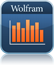Wolfram Statistics Course Assistant is available for a couple of dollars.  Look for the title on your preferred device: iOS, Android, or Windows.  See it on the Web.  Per the description, this app enables you to create a bar chart, histogram, or scatter plot of any set of data; find the mean, median, mode, standard deviation, quartiles, and interquartile range of a dataset; calculate normal probabilities and find information about the normal distribution; calculate binomial probabilities and find information about the binomial distribution; compute probabilities based on dice rolls and coin flips; find the best-fit line of a set of data points; select random integers or random real numbers.

### Teach using data simulations for better understanding of statistics and statistical reasoning.Data simulations can help learners answer particular research questions or to solve statistical problems.  Get more information on how to teach using data simulations at Teaching with Data Simulations, which is within Pedagogy in Action, the SERC portal for Educators.  Examples are included.  Use simulation software such as Fathom Dynamic Data software, which allows you to create simulations.

Web applets are also beneficial.  Consider:

See CT4ME's Math Manipulatives for other possibilities for exploring statistics and other subjects.

#### Help students to interpret data visualizations.What's Going On in This Graph? from The New York Times Learning Network and the American Statistical Association is a free online weekly feature meant for learners in grades 7-12+.  The Times posts graphs, charts, and maps and invites students to discuss them live with statistics teachers who moderate student comments.  These are valuable to help students gain statistical literacy and learn to read, interpret, and question data--a key 21st century skill.  The schedule is posted online.  Resources include a list of past graphs.

## Multiple Subject SitesAnalyze Math contains math tutorials, problems, and worksheets for various topics in algebra, trig, geometry, precalculus, calculus, statistics, and more.

CK-12 Foundation is a non-profit organization with a mission to reduce the cost of textbook materials for the K-12 market both in the U.S. and worldwide.  In addition to its Flexbooks, there are online problem sets in multiple math subject areas.  There is a section for elementary math for grades 1-5 aligned to the Common Core standards.  It contains interactive math practice problems, videos, video hints, and full step-by-step solutions, all free.  Other sections include arithmetic for middle school, algebra, geometry, probability, statistics, trigonometry, analysis, and calculus.  Also see the CK-12 Foundation Dedicated Channel for videos on YouTube.  CK-12's standards-aligned digital math flexbooks for middle and high school (including calculus) are customizable.CoolMath.com has lessons in pre-algebra, algebra, pre-calculus, and more.

HOT!: DeltaMath includes over 1800 premade problem types for grades 6, 7, and 8, algebra 1, geometry, algebra 2, pre-calculus (including trigonometry topics) and calculus.  The free teacher account features unlimited student practice problems and assignments, autograding, detailed student data, including time-stamps, evidence of student progress/learning, cheat prevention tools, copy and share assignments, and Google single sign-on.  A strong feature is that step-by-step solutions are provided when a problem is incorrectly answered.  Resources can be sorted by Common Core standards.  Paid versions include additional features such as videos, and integration with Google classroom, Clever or ClassLink.

edX, founded by Harvard and MIT, enables learners to take "free online math courses from MIT, ASU, and other leading math and science institutions. Get introductions to algebra, geometry, trigonometry, precalculus and calculus or get help with current math coursework and AP exam preparation."  You'll also find courses in pre-algebra, logic,  probability, statistics, regression, and linear algebra.Electronic Bookshelf is an outgrowth of the original project developed at Dartmouth College called Math Across the Curriculum (MATC).  Now part of the Center for Mathematics and Quantitative Education at Dartmouth, it features materials with an interdisciplinary approach to mathematics topics.  Among topics in the bookshelf topics are algebra, calculus and differential equations, physical science, art, computer sciences, pre-algebra, history and geography, literature and writing, biology/medicine/psychology, data analysis, statistics, business/economics, engineering, math and music, financial literacy, health QL, and discrete math.  There's a little bookshelf of K-12 resources.

Flippedmath.com promotes "Flipped Mastery Learning: Mathematics Without Boundaries."  Free online courses in Algebra 1 (Common Core and Traditional), Geometry, Algebra 2 (Common Core and Traditional), Pre-Calculus, and AP Calculus include multiple videos.  They were developed to "allow students to demonstrate mastery of all concepts and progress at their individual pace."  Teacher resources include How Flipped-Mastery Works.GeoGebra is free, open-source, dynamic mathematics software that joins geometry, algebra, tables, graphing, statistics, and calculus.  You can do constructions with points, vectors, segments, lines, conic sections as well as functions and change them dynamically afterwards.  You can enter equations and coordinates directly. GeoGebra has the ability to deal with variables for numbers, vectors and points, finds derivatives and integrals of functions and offers commands like Root or Extremum.  It received several international awards, including the European and German educational software awards. In Dynamic Mathematics with GeoGebra, authors Markus Hohenwarter, principle developer of the software, and Judith Preiner (2007) said, "You can use GeoGebra both as a teaching tool and to create interactive web pages for students from middle school up to college level. Specifically designed for educational purposes, GeoGebra can help you to foster experimental, problem-oriented and discovery learning of mathematics" (Abstract section).  They illustrate the basic ideas of the software and some of its versatile possibilities by discussing several interactive examples.  Visit GeoGebra's Blog.  GeoGebra also has a "Materials" section where you can also share and find materials for arithmetic, geometry, trigonometry, calculus, probability, algebra, functions, and statistics, and activities specific for elementary, middle, and high school.

Google: Exploring Computational Thinking (CT) contains classroom-ready lessons, worksheets, and examples illustrating how educators can incorporate CT into the K-12 curriculum. You'll find everyday applications, too, such as pattern recognition, algorithm design, and other computational thinking skills.  Content was developed by teachers in collaboration with Google software engineers; math addresses pre-algebra, algebra, geometry, and calculus.  Additional features include a Getting Started Guide, web resources, and a discussion forum to connect with other CT educators.  Readers might be particularly interested in the conceptual frameworks and how-to papers listed in web resources for incorporating CT into every classroom.HippoCampus is an open resource for personalized learning, which offers free educational resources for middle school through college.  Multimedia content includes videos, animation, and simulations in multiple subjects.  Math includes arithmetic, algebra and geometry, calculus and advanced math, statistics and probability.  Users do not need to register or sign-in to use the site.  Content provided at HippoCampus is created by other institutions and contributed to the site for distribution.  The site is powered by the NROC Project.  Individual instructors can create custom playlists to better meet the needs for groups of students.Interactive Mathematics promotes learning math while you play with it!  Primarily for grade 8 algebra through upper secondary and college level students; math lessons include topics in algebra, complex numbers, counting & probability, logarithms, trigonometry, money math, plane analytic geometry, and graphing.  Calculus includes differentiation, integration, and higher topics in calculus.  Lectures and full examples are provided.  There's also a section on math art in code.  A complete list of interactive graphs, calculators, and other manipulatives is available.  The site is the work of Murray Bourne.

Khan Academy offers K-8 and high school math courses with videos and practice problems.

Kopsas Math Tutorials includes an extensive collection of videos for algebra, trigonometry, calculus, geometry, probability & statistics created by Daniel Kopsas of the Ozarks Community College.

Mangahigh is devoted to mathematics game-based learning for middle and high school learners.  Games are of a commercial quality, but are free.  You'll find interactivity, more than one level of difficulty, and topics such as transformations, estimation with basic number calculations, order of operations, equation solving (linear, quadratic, cubic, plotting coordinates), basic shapes to advanced geometry (some trig, too), ordering of decimals, fractions, percents.  Adaptive learning, which automatically adjusts difficulty level based on how students engage with the game, is featured in Prodigi where students work to master skills in number, algebra, data, and shape.  Games are endorsed by well-known UK mathematician Marcus du Sautoy.

MathBits.com "is devoted to offering fun, yet challenging, lessons and activities in secondary (and college level) mathematics and computer programming for students and teachers."  Subjects include algebra 1, geometry (including materials for using Geometer's Sketchpad), and algebra 2, principally.

MathFLIX from Loyola University Chicago School of Education is a real find.  It has 1000 free "instructional math movies covering a wide range of math concepts including Number & Operations, Algebra, Measurement, Geometry, Data Analysis & Probability, Connections and Technology. In addition to MathFLIX’s valuable video resources, the site also features 400 downloadable worksheets that reinforce concepts and provide valuable practice."  Each movie is from 4-7 minutes long.Math Is Fun provides supplementary content for K-12 in topics ranging from working with numbers and basic operations to money, algebra (with an introduction to trigonometry), geometry (including some interactives for constructions and using a protractor), data, measurement, worksheets, games, puzzles, and an illustrated math dictionary.  Tutorial information is provided within content.

Mathispower4u by James Sousa contains a collection of over 6000 mini-lessons and videos organized by course and topic: number sense, arithmetic/prealgebra, algebra 1, algebra 2, geometry, math for liberal arts, trigonometry, calculus 1, calculus 2, calculus 3, linear algebra, differential equations.  The site also includes problem solving activities with video solutions.  Multiple Common Core topics are addressed in these.

Math Expression has excellent tutorials, including animated videos with audio explanations and text equivalents.  Topics include graphing (e.g., using the coordinate plane, slope, writing equations of lines), basic algebra (e.g., variables, expressions, solving equations), exponents, fractions, symmetry, and volume.  Math study tips and practice questions help reinforce concept development.  There is also a Q&A Library.  The site is by Wei Chong, and is well-worth investigating.

HOT!: Math Portal Online Calculators and Solvers by mathematician Miloš Petrović contains multiple math calculators and solvers grouped into 17 categories.  Use this site for problems including polynomial equations, rational expressions, systems of equations, vectors, matrices, complex numbers, plane and analytic geometry, statistics, trigonometry, calculus (limits, derivatives, integrals), sequences, series, financial, numbers, and more.  Per the description, "These calculators can help you with basic and advanced problems in high school and college math.  All of them are capable of performing exact computations.  Also, almost every of them will generate a step by step explanation."  The step by step explanations are exceptionally valuable for learners.  There are three other math sections on the site: formulas, tests and lessons.  Math lessons relate to algebra, calculus, analytic geometry, and linear algebra, and also include solved problems and examples for students to solve.

Math Warehouse has numerous animations, lessons, and interactive practice problems for algebra, geometry, trigonometry, and calculus.  There is also a collection of worksheets.

HOT!: New Jersey Center for Teaching and Learning: Course Materials include free STEM resources for K-12, including advanced level courses.  Mathematics courses for high school include algebra I and II, geometry, pre-calculus, AP Calculus at AB and BC levels, and Integrated Math levels I, II, and III.  They are also available in Spanish.  You'll find presentations, classwork-homework assignments (includes answers), lab activities, and assessments in unit resources, as appropriate for each course.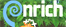NRICH from the University of Cambridge is devoted to enriching mathematics for learners in grades 1-12 with games, problems, investigations, articles and books.  Resources are free.  The collection of activities for topics in secondary mathematics are designed to develop subject matter knowledge and problem solving skills.  These are organized by number, algebra, geometry and measures, handling data (statistics and probability), thinking mathematically.  You'll find articles for developing mathematical habits of mind, and a topic for advanced mathematics (e.g., calculus, advanced probability and statistics).  There's a post 16 section for learners 16 and older. Sections for primary and secondary teachers include professional development.

OER Commons lists over 7000 open education resources for math, searchable by subject, standard, grade level, learning domain.

PatrickJMT's Math Channel on YouTube contains an extensive collection of his videos for arithmetic, algebra, linear algebra, trigonometry, differential equations, discrete math, probability and statistics, calculus, and more.  They are free to use and are suitable for high school and above.

PBS LearningMedia includes free media resources searchable by grade level, standards, media type, and subject. Resources include video and audio segments, interactives, images, documents, lesson plans, productivity tools for teachers, self-paced lessons,and student-oriented activities. You'll find media from NOVA, Frontline, American Experience, and other public broadcasting and content partners.  The mathematics section features K-8 mathematics strands, and high school number and quantity, algebra, geometry, functions, statistics and probability.  Highly recommended.

Softschools.com provides free math worksheets, games, and online practice activities/quizzes for preK-5, middle school, and high school (e.g., algebra 1 and 2, geometry, some trig and calculus) in multiple concepts.  These are organized by grade, subject, and topic.  Explanations of many concepts are provided.

S.O.S. Math from MathMedics has over 2,500 pages of short explanations in multiple subjects: algebra, trigonometry, calculus, differential equations, complex variables, matrix algebra, or mathematical tables.  There's also a section for test prep: intermediate algebra, mathematics for the social sciences (I and II), precalculus (I and II), calculus, and differential equations.

Study.com has online math courses in pre-algebra, algebra, geometry, statistics, precalculus, calculus, college math and more.  These feature video lessons from experienced educators, transcripts of those lessons, supplementary content, quizzes, and exams.  Learners can potentially earn college credit.

The Math Page is by Lawrence Spector of the Borough of Manhattan Community College, The City University of New York.  This site has several major sections.  Practice problems illustrating concepts are presented throughout with answers.

• Skills in Arithmetic is a complete course, which includes addition, subtraction, multiplication, division; mental arithmetic, powers of 10, regrouping, decimals, parts of natural numbers, fractions, and percents.
• Plane Geometry
• Topics in Trigonometry addresses ratio and proportion, definitions of the trig functions, solving right triangles, the 30-60-90 degree right triangle, the law of sines, the law of cosines, the unit circle, radian measure, and arc length.
• Topics in Precalculus include formal rules of algebra, rational and irrational numbers, functions and graphs, logarithms, the binomial theorem, and mathematical induction.
• An Approach to Calculus
• The Evolution of Real Numbers.

Walter Fendt's HTML5 Applets on Mathematics address arithmetic, plane geometry, trigonometry (visual demonstration of graphs of sine, cosine and tangent of an angle), vector calculation, analysis (e.g. app to introduce the derivative, 1st and 2nd derivatives), and complex numbers (arithmetic operations).  These are very engaging and illustrative of concepts.

Zona Land's More Mathematics than Science is devoted to helping students understand algebra, geometry (including conic sections), trigonometry, and curve fitting.  Don't miss the interactive manipulatives and virtual reality demonstrations of concepts.

## Spice up your math class!

SPELL YOUR NAME IN MATH!  Get your own personal polynomial at the Global Math Project and then learn about the math that generates your name.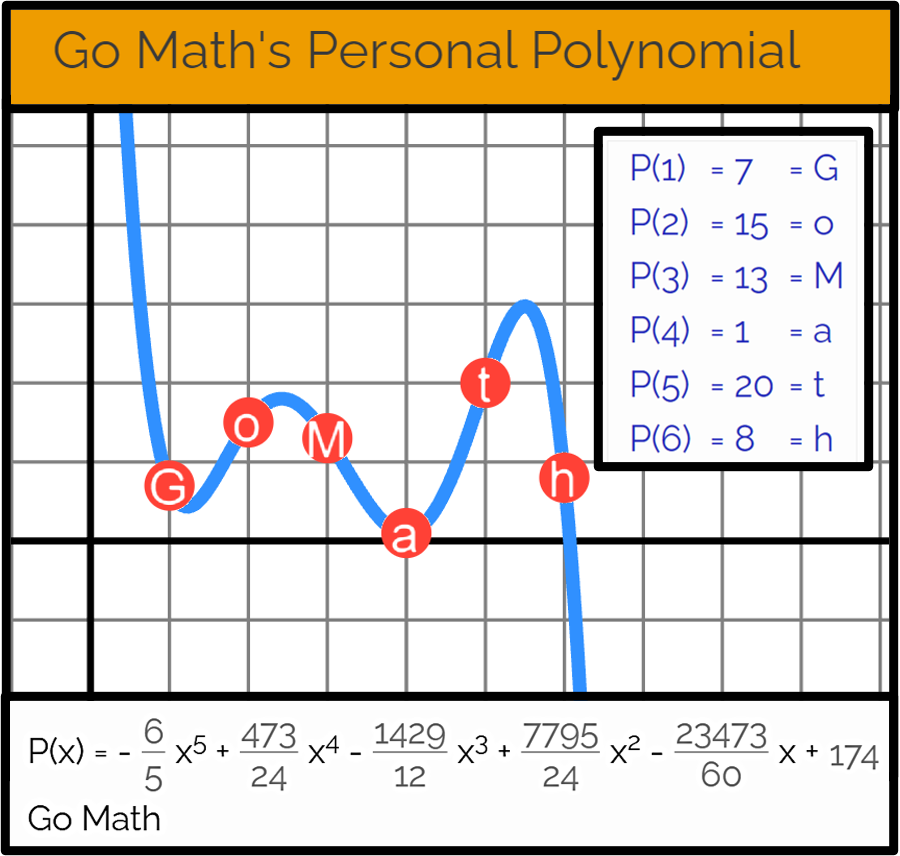Mudd Math Fun Facts.  Fun Facts are designed for all areas of mathematics, and work best in courses where the typical student needs that boost to generate interest and arouse curiosity in math.  The Fun Facts will cause students who are studying algebra, geometry, probability, calculus, number theory, and topology, for example, to look at mathematics differently!  Per its author, Francis Su,"Fun Facts give students a glimpse that mathematics is full of interesting ideas, patterns, and new modes of thinking" (About section).  See the list of All Fun Facts.Taken from the quick activity on multiplication by 11, can you figure out what's going on here?

253 x 11 = 2783

117 x 11 = 1287

532 x 11 = 5852

267 x 11 = 2937

#### Did you know that an ellipse is just a flattened circle?

Examine the formula for the area of a circle: A=π r2.  Do you see that the ellipse area is A=π a b ?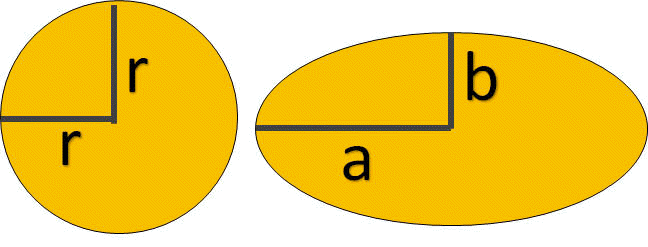Spice up your class with Larry Gonick's The Cartoon Guide to Algebra (2015), which covers all the algebra essentials. Demystify first-year calculus as your students delve into functions, limits, derivatives, and integrals.  The Cartoon Guide to Calculus (2011), also by Larry Gonick, is just what you need. Coauthored by Grady Klein and Alan Dabney, The Cartoon Introduction to Statistics (2013) adds humor to your introductory statistics course.

#### How about presenting a number of the day, or a math question or riddle of the day or a brain teaser?

MathsStarters: Number of the Day

Mathopolis features a Mathematics Question of the Day for each grade 2-8 and high school geometry, algebra 1, algebra 2, statistics, and calculus.  Each has a worked solution.  These are great classroom starters.

Good Riddles Now GRiN:) has an entire section devoted to math riddles with their answers.  "All of these tricky riddles are based on real math concepts and can be solved with purely math and logic."

SkyGaze.com has Interesting Facts on Math and Numbers.  The site also features other interesting facts, strange and unexplained topics and events, and mysteries and secrets.

Paige Tutt (2023, July 28) posted a list of 10 Brilliant Math Brain Teasers at Edutopia.  These are suitable for middle and high school learners.  Each is a great way to start a lesson.  Also see the 35 math brain teasers for kids by Jill Staake (2022, July 14) at WeAreTeachers.

#### Or spice up your lesson with video.WatchKnowLearn.org contains an extensive directory of subject topics.  The database of free educational videos for math (and other subjects) is organized by topic area and subcategories within each of those.  There are about 5000 videos in mathematics, and over 200 on standardized test skills and math study skills.  Videos are gathered from across the internet and are previewed by a team of professionals.  Teachers will find the database helpful, too, to get new ideas on how to approach material and videos can be used in their classrooms.  Highly recommended site!Explore numbers and shapes, applied math for young learners, arithmetic, measurement,  algebra, geometry, precalculus, and calculus, the history of math, and more.

#### How about presenting a math joke, comic of the day, a math song or a math rap?

Let your students also create their own.  Do some doodles.

Per Joan Boaler (2016) and colleagues at Stanford University,"Good mathematics teachers typically use visuals, manipulatives and motion to enhance students’ understanding of mathematical concepts" (p. 1).  "Drawing mathematical ideas helps mathematics users of any level, including mathematicians, to formulate ideas and develop understandings."  Students can represent their ideas in "a multitude of ways, such as through pictures, models, graphs, even doodles ... or cartoons" (p. 5).

Jokes?  Prodigy contains 101 Silly Math Jokes and Puns to Make Students Laugh Like Crazy.

Doodles? See an extensive list of possibilities for Doodling in Math Class, posted on YouTube.

HOT!: MakeBeliefsComix by Bill Zimmerman allows anyone to create comic strips online for free, then print or email them.  Students might create a comic to explain a math concept or a new vocabulary word, or how they solved a problem.  They might share their feelings or a concern about their learning.  It's a fun way to incorporate writing in math class.  The site contains several features to help create a comic, such as backgrounds, balloons and prompts, characters, comic strip starters with writing prompts, a teacher section, help 4 students, and more.

Pixton is a social cartoon making website that anyone can join. The site includes safety tips, tips for parents, and a special section for school use.  A site search reveals many math cartoons. You can leave comments, rate your favorites, exchange messages with friends, build a fanclub, print and download your own comics, email them to others, and post them on other web sites and blogs.

The Rappin' Mathematician explains How to Write Your Own Math Rap.  Need an example?  Visit his website: https://alexkajitani.com/the-rappin-mathematician/ which contains a few free raps.

Songs for Teaching includes Math Songs: Teaching Math Facts & Concepts.  Songs are categorized by addition, subtraction, multiplication, division, advanced math, algebra, geometry, trigonometry, and there is a section for miscellaneous math songs.

Storyboard That is an award-winning drag-and-drop tool to create visuals for teaching, learning, and communicating.  The site offers a free version and a paid subscription version.  The storyboard creator offers multiple scenes, characters, textables, infographics, web and wire frames, science icons, worksheet formats, and more.  There is a teacher resource section with examples, lesson plans and activities for elementary, middle, and high school.

See Common Sense Education for a list of classroom-friendly websites and apps for making comics.

Culturally Situated Design Tools for teaching math and computing through culture comes with lessons and software for students to create their own cultural designs associated with African, African American, Latino, Native American cultures, and Youth Subcultures—even graffiti!

#### You could present a mathematician of the day or introduce the history of mathematics to your learners.

A Brief History of Mathematics hosted by the BBC in the UK contains a series of 10 podcasts in which Professor of Mathematics Marcus du Sautoy "reveals the personalities behind the calculations and argues that mathematics is the driving force behind modern science."  You'll learn about contributions of mathematicians and challenges they faced.  Those featured include Bourbaki, Hardy and Ramanujan, Poincare, Cantor, mathematicians who helped Einstein; Gauss, Galois, Fourier, Euler, Newton and Leibniz.  Each podcast is about 15 minutes.Egyptian Mathematics Numbers Hieroglyphs also includes a history of ancient Egypt.  Imagine writing your numbers with seven different symbols: a single stroke, a hobble for cattle, a coil of rope, a lotus plant, a finger, a tadpole or frog, or a god with his arms raised above his head.  Read more on this topic from Mark Millmore and explore doing a few problems with Egyptian Math.The Two Hundred Greatest Mathematicians of All Time including some from the 20th century created by James Dow Allen.  Get some facts on mathematicians such as Newton, Archimedes, Euclid, Gauss, Pythagoras, Lagrange, Fermat, Descartes, Leibniz, Hilbert, and more.

Mathigon's Timeline of Mathematics features the contributions of famous mathematicians from Thales in 600 BC to the present day.

Math Matters includes a brief look at how mathematics has influenced modern life.  It highlights the top twenty technological advances of the twentieth century, and explores the role of mathematics in bringing these innovations to reality.  Consider electrification, highways, electronics, water supply and distribution, household appliances, petroleum and petrochemicals, airplanes, spacecraft, high performance materials, air conditioning and refrigeration, nuclear technology, radio and TV, computers, imagining, telephones, the internet, health technologies, agricultural mechanization, laser and fiber optics, and automobiles.  The events and historical influencers are online and a downloadable poster is available.

The Mathematical Association of America: On This Day is a section of the site where you can click on any day to learn about a selection of historical events related to mathematics that occurred on that day.The MacTutor History of Mathematics of the University of St. Andrews in Scotland contains an extensive collection of  biographies, history topics (e.g., mathematics in various cultures and mathematics subject area topics), chronologies, mathematicians of the day, famous curves, quotations from many of the mathematicians, and more.  So, you can check this site daily.

Biographies of Women Mathematicians is a project from Agnes Scott College in Atlanta, Georgia.  The achievements of women in mathematics are sure to motivate.  You also will find the woman mathematician of the current day.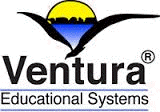Ventura Educational Systems has several apps for iPad and Apple TV featuring the Giants in Math and Science.  Learn about the contributions and lives of Ada Lovelace, Albert Einstein, Blaise Pascal, Euclid, Leonard Fibonacci, Carl Gauss, Isaac Newton, Pythagoras, René Descartes, and others.  The apps are interactive learning tools with graphical illustrations.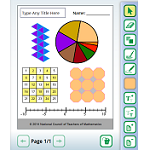Dynamic Paper is a free applet from NCTM Illuminations.  With it you can design and print the following, as stated in the app instructions:

• Nets – two-dimensional outlines of three-dimensional shapes, including regular polyhedra, prisms, pyramids, cylinders and cones
• Graph Paper – coordinate graphs, polar coordinates, logarithmic graph paper
• Number Lines – including positive and negative coordinates
• Number Grids – hundreds boards and the like
• Tessellations – tiling patterns involving triangles, quadrilaterals, and hexagons
• Shapes – pattern blocks, attribute blocks, and color tiles
• Spinners – up to 16 sectors, with adjustable sizesGraph paper and grids from Mathbits.com: number lines, dot, rectangular, trig, polar, and blank calculator screens---over 30 different types.Print free graph paper! -- Cartesian, polar, logarithmic, and more.

Print graph paper of all kinds from Incompetech.com: square, triangle, rhombus, hexagon, octagon, trapezoid, circular, asymmetric, accounting, log, polar, number line, storyboard, perspective, note-taking, and so on.  A terrific collection.

Printable Paper includes more than Cartesian, polar, and logarithmic graph paper.  There's pentagon, hexagon, octagon graph paper, 3D graph paper, and paper templates in other areas beyond math.

#### References

Boaler, J., Chen, L., Williams, C., & Cordero, M. (2016). Seeing as understanding: The importance of visual mathematics for our brain and learning. Journal of Applied & Computational Mathematics, 5(5). doi: 10.4172/2168-9679.1000325. https://www.youcubed.org/wp-content/uploads/2017/03/Visual-Math-Paper-vF.pdf

Hohenwarter, M., & Preiner, J. (2007, March). Dynamic mathematics with GeoGebra [online]. Journal of Online Mathematics and Its Applications, 7, article 1448. https://www.maa.org/node/115964

National Mathematics Advisory Panel (2008). Foundations for success: The final report of the National Mathematics Advisory Panel.  Washington, DC: U.S. Department of Education. https://files.eric.ed.gov/fulltext/ED500486.pdfBack to top | Math Resources: Page  1  |   2   |   3   |   4   |   5   |   6See related topics:  Math Manipulatives and Standardized Test Preparation.